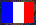Multiplication by Integer Constants

Introduction

This page deals with the problem of performing multiplications by integer constants using elementary operations (e.g. left shifts by any number of bits, corresponding to multiplications by fixed powers of two, additions and subtractions), and in particular, algorithms generating such codes. These algorithms can be used either directly by the programmer (for instance in multiple precision, for the Toom-Cook-like algorithms to multiply large multiple-precision integers and for the approximate computation of consecutive values of a polynomial) or by compilers to generate integer multiplications for some processors. The generated code can be implemented either in software or in hardware (e.g. on FPGAs). The main problem is to obtain a code as good as possible in a reasonable time (for instance, in a polynomial time).

Papers

My main papers on this subject, in chronological order...

• [Lef1999b] Multiplication by an integer constant, LIP research report RR1999-06, January 1999.

This research report describes my first algorithm based on a search for repeating patterns in the binary representation of the constant. Note that the conjecture in Section 5.3 is false (this is a consequence of results in my paper on lower bounds on the length of the generated code); this also shows the irregularity of the problem.

• [DinLef2000a] Constant multipliers for FPGAs (written with Florent de Dinechin), in the Second International Workshop on Engineering of Reconfigurable Hardware/Software Objects (ENREGLE 2000), June 2000. Also available as LIP research report RR2000-18.

The application to the FPGAs...

• [Lef2001b] Multiplication par une constante, in Réseaux et Systèmes Répartis, Calculateurs Parallèles, 2001. A slightly older version is available in English as INRIA research report RR-4192.

This article describes an improvement of my first pattern-based algorithm, in particular; in fact, it is a natural extension of this algorithm to multiply a same number by several constants (as in a multiplication of a vector by a constant matrix). The various algorithms described in this paper are compared with each other, and also with what could be obtained with an algorithm generating optimal code.

• [Lef2003a] Multiplication by an integer constant: lower bounds on the code length, in the RNC'5 proceedings, September 2003.

This paper is more theoretical than the previous ones. Using results from the theory of information, we look for (mainly asymptotic) lower bounds on the length of a code of a multiplication by a constant (obtained either by any algorithm, which corresponds to the optimal case, or by a given algorithm). A study on the maximal shift count in an optimal code is also carried out.

In this paper, I gave a class of optimal programs for which the asymptotic ratio of the maximum shift count over the constant size is 7 / 6. I later found a similar example (and a similar proof) leading to the asymptotic ratio 3 / 2: For h ≥ 4, consider n = (1 + 2h)  (1 + 2h + 2)  (1 + 2h + 4) − 23h + 6, which is a (2h + 7)-bit integer (22h + 6 + 22h + 4 + 22h + 2 + 2h + 4 + 2h + 2 + 2h + 1) that can be computed with an optimal program (4 elementary operations) using a shift count of 3h + 6.

I no longer work on this subject (at least currently). Other people have worked on it since, cf Section See Also.

Software

bernstein.c

My implementation in ISO C of Bernstein's algorithm.

patterns

My implementation in Perl of my algorithms based on repeating patterns; it has a good time complexity, but uses much memory, as almost everything is cached. Run this script without arguments for some documentation on its usage. Note: I wrote this Perl script mainly for my own research work, not as a software that can be used by anyone. It is distributed under the GNU General Public License (version 2 or later).

[2015-08] New: Mode m is threaded. Some results with my common subpatterns algorithm (mode m, i.e. exhaustive tests); results for 28 ≤ n ≤ 37 were obtained in August and September 2015.

rigo.c

The implementation in C (using GMP) of my latest algorithm, by Raphaël Rigo. It is distributed under the GNU General Public License (version 2 or later).

MulByConst.tar.xz

My old source files with their RCS history, written between 2000 and 2003. The main goal of this archive is to be able to check the codes used for some of my articles.